## For a double slit, as the slit separation is increased, what happens to the pattern? Does the angle to the first diffraction minimum increas

Question

For a double slit, as the slit separation is increased, what happens to the pattern? Does the angle to the first diffraction minimum increase or decrease or stay the same? Does the angle to the first interference maximum increase or decrease or stay the same?

in progress 0
2 months 2021-07-21T10:27:02+00:00 1 Answers 2 views 0

As slit separation (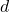) is increased, angle of first diffraction minimum will remains same.

As slit separation () is increased, angle to the first interference maximum is decrease

Explanation:

Given:

From the formula of interference and diffraction,

⇒ For diffraction,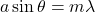Where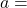width of slit,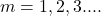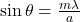⇒ For interference,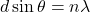Where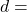distance between two slit,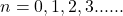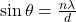As slit separation () is increased, angle of first diffraction minimum will remains same.

As slit separation () is increased, angle to the first interference maximum is decrease